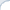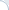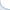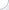Last week I shared my time between work and hacking at a forth machine translation marathon in the Americas. This event organized jointly by CMU and Amazon (sponsored by Amazon) was a lot of fun. My small sub-team of two people got familiar with OpenNMT, trained English-German and English-Ukrainian models, as well as implemented an idea of our team lead Adithya Renduchintala. Hey, we have even gotten a tiny 0.5 gain in BLEU for the English-Ukrainian pair!

We certainly learned a lot of lessons, most of which generalize well beyond the neural machine translation task. One is related to implementation of custom neural modules and PyTorch. Unlike TensorFlow and many other packages, PyTorch belongs to a new crop of neural frameworks, where a neural network (computation) graph is dynamic. What does it mean? It means that you do not have define a computation graph in advance. You can simply write a tensor-manipulating code and PyTorch will do all the back-propagation and parameter updating automatically. Another well-known package with a similar functionality is DyNet.

There are ups and downs to dynamic computation graphs. For one thing, it is much simpler to debug them. For another, there is a lot of magic going behind the scenes, which you need to understand. First of all, one needs to remember that the computation graph is defined by a sequence of manipulations on Tensors and Variables (Variable is a Tensor wrapper that got deprecated in the recent PyTorch). Your sequences should be valid and properly linked so that all the Tensors of interest have a chance to be updated during back-propagation.

Tensor-level manipulations can easily get hairy. To simplify things, PyTorch introduces an abstraction layer called Module. A Module is a basic building block that has some parameters and a function forward to turn inputs to outputs. If all is done properly, given only the forward function PyTorch can compute the gradients via back-propagation and update the model parameters. The nice thing about PyTorch is that you can easily write a new module by combining several existing ones. There is no need to write arcane description of layers! As we can see from this PyTorch example, we can define a forward network computation in a very straightforward way. Even if you have not seen a line of PyTorch code before, you can easily figure out that this module applies two 2d-convolutions each of which is followed by a RELU non-linearity:

class Model(nn.Module):    def __init__(self):        super(Model, self).__init__()        self.conv1 = nn.Conv2d(1, 20, 5)        self.conv2 = nn.Conv2d(20, 20, 5)     def forward(self, x):        x = F.relu(self.conv1(x))        return F.relu(self.conv2(x))

But here is a catch that is barely mentioned in PyTorch documentation. Although writing the forward function is sufficient to compute the gradients, it is apparently not sufficient to determine which tensors represent module's parameters. In the above example, the module includes two convolutional neural networks, each of which has parameters to be updated. How does PyTorch know this? Well, turns out that PyTorch overloads the function __setattr__! Thus, it surreptitiously "registers" each submodule when a user makes an assignment like this one:

self.conv1 = nn.Conv2d(1, 20, 5)

Unfortunately, such automatic registration does not work all the time. Imagine, for example, you want to aggregate several sub-modules whose number is not known in advance. It is very natural to save them all in a list:

class Model(nn.Module):    def __init__(self):        super(Model, self).__init__()        self.sub_modules = []        self.sub_modules.append(nn.Conv2d(1, 20, 5))  # append a module to the list        self.sub_modules.append(nn.Conv2d(20, 20, 5)) # append a module to the list

Yet, this is where PyTorch magic stops: If you place the modules in plain Python list, PyTorch will not be able to update their parameters. As a fix, you need to register them explicitly. One way to do this is to explicitly call the function add_module. A less tedious ways is to use a combination of nn.ModuleList and nn.Sequential. Please, read a discussion here for more details.